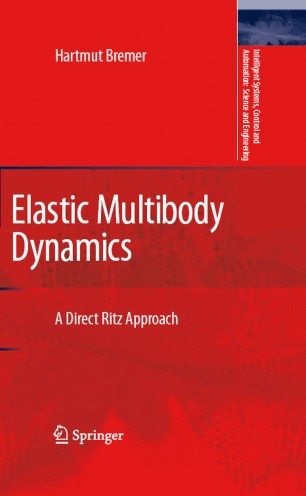# Elastic Multibody Dynamics

## A Direct Ritz Approach

• H. BremerBook

1. Front Matter
Pages i-ix
2. Pages 1-6
3. Pages 7-27
4. Pages 29-58
5. Pages 59-114
6. Pages 383-429
7. Back Matter
Pages 431-449

### Introduction

This textbook is an introduction to and exploration of a number of core topics in the field of applied mechanics:

On the basis of Lagrange's Principle, a Central Equation of Dynamics is presented which yields a unified view on existing methods. From these, the Projection Equation is selected for the derivation of the motion equations of holonomic and of non-holonomic systems.

The method is applied to rigid multibody systems where the rigid body is defined such that, by relaxation of the rigidity constraints, one can directly proceed to elastic bodies. A decomposition into subsystems leads to a minimal representation and to a recursive representation, respectively, of the equations of motion.

Applied to elastic multibody systems one obtains, along with the use of spatial operators,

a straight-on procedure for the interconnected partial and ordinary differential equations and the corresponding boundary conditions. The spatial operators are eventually applied to a RITZ series for approximation. The resulting equations then appear in the same structure as in rigid multibody systems.

The main emphasis is laid on methodical as well as on (graduate level) educational aspects. The text is accompanied by a large number of examples and applications, e.g., from rotor dynamics and robotics. The mathematical prerequisites are subsumed in a short excursion into stability and control.

### Keywords

Central Equation of Mechanics/Mechatronics Direct RITZ approach Operators and recursive algorithms Partial and ordinary differential equations of motion Potential Projection Equation Rotation Rotor Torsion mechanics modeling partial differential equation robot robotics stability

#### Authors and affiliations

• H. Bremer
• 1
1. 1.Johannes Kepler UniversityAustria